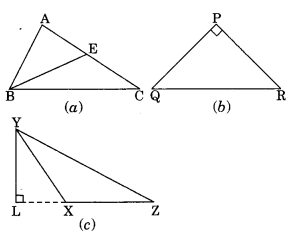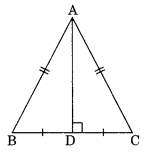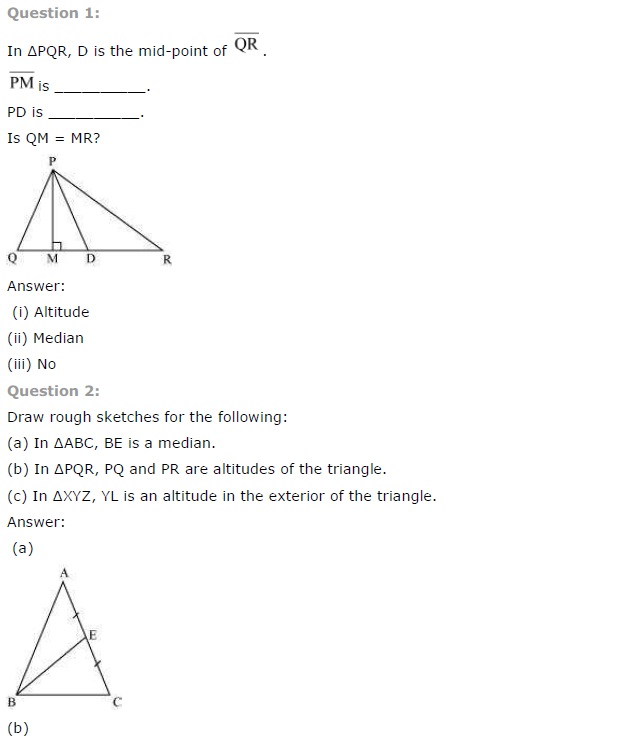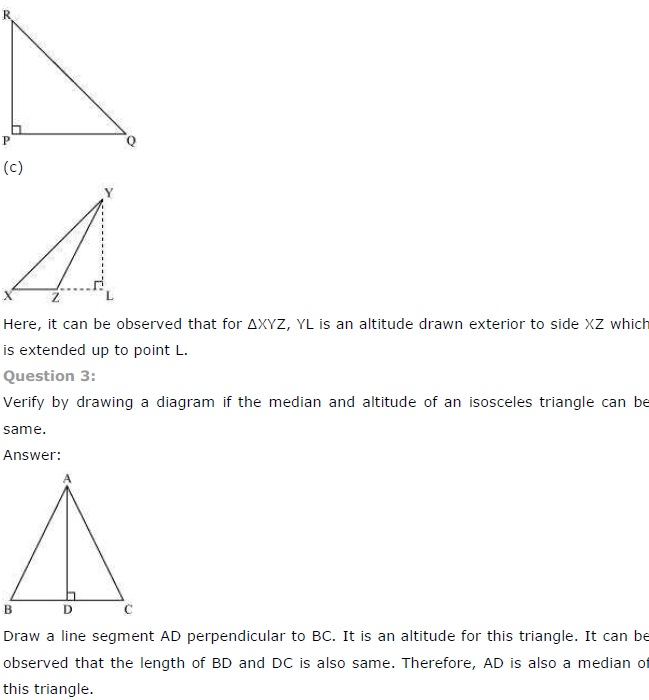# NCERT Solutions for Class 7 Maths Chapter 6 The Triangle and its Properties

NCERT Solutions for Class 7 Maths Chapter 6 The Triangle and its Properties

### NCERT Solutions for Class 7 Maths Chapter 6 The Triangle and its Properties Ex 6.1

NCERT Solutions for Class 7 Maths Chapter 6 The Triangle and its Properties Exercise 6.1
Ex 6.1 Class 7 Maths Question 1.If QM = MR?
Solution:Ex 6.1 Class 7 Maths Question 2.
Draw rough sketches for the following:
(a) In ∆ABC, BE is a median.
(ib) In ∆PQR, PQ and PR are altitudes of the triangle.
(c) In ∆XYZ, YL is an altitude in the exterior of the triangle.
Solution:Ex 6.1 Class 7 Maths Question 3.
Verify by drawing a diagram if the median and altitude of an isosceles triangle can be same.
Solution:
∆ABC is an isosceles triangle in which AB = ACDraw AD as the median of the triangle.
Measure the angle ADC with the help of protractor we find, ∠ADC = 90°
Thus, AD is the median as well as the altitude of the ∆ABC. Hence Verified.## SabDekho

The Complete Educational Website